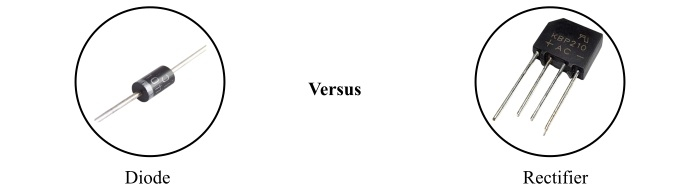# Difference between Diode and Rectifier

The terms diode and rectifier are extensively used in electronics engineering. Sometimes, both these terms seam similar because they perform similar functions. However, there are many differences between a diode and a rectifier.This article is meant for explaining the differences between diode and rectifier. But before discussing the differences, it is advantageous to learn the basics of these two devices so it becomes easier to understand the differences between them.

## What is a Diode?

A diode is a two terminal semiconductor device that allows the flow of electric current only in one direction. Therefore, the diode can function as a static switch that can control the flow of current in an electric circuit.

The different types of diodes are as: signal diode, rectifier diode, power diode, photodiode, Zener diode, Schottky diode, etc.

A diode has two terminals namely anode and cathode. When a voltage is applied to the diode such that the positive terminal of the source is connected to the anode terminal and negative terminal to the cathode terminal, then the diode is called forward biased and allow the flow of current.

On the other hand, if the positive terminal is connected to cathode and the negative terminal to the anode, then the diode is said to be reverse biased and stops the current flow through it.

A most extensive use of a diode is for the conversion of alternating current (AC) into direct current (DC). Other applications of diode are in signal limiters, static switches, oscillators, voltage regulators, etc.

## What is a Rectifier?

A rectifier is an electronic circuit that can convert an alternating current (AC) into direct current (DC). A rectifier uses a solid state electronic switching device such as diode, SCR, etc. as its main component.

A rectifier receives an alternating current of specified voltage as input and converts it into a pulsating DC at the output. Then, this pulsating DC is filtered to obtain a constant DC by employing a filter circuit.

Based on the semiconductor device used for switching in the circuit, the rectifier may be of following two types,

• Uncontrolled rectifier
• Controlled rectifier

The uncontrolled rectifier is the circuit which uses diode as the main component for rectification. It is so called because the operation of the diode cannot be controlled as desired. On the other hand, a controlled rectifier uses an SCR (silicon controlled rectifier) as the main circuit component. A controlled rectifier is so called because the operation of the SCR can be controlled according to requirement with the help of a gate signal.

## Difference between Diode and Rectifier

Though the primary function of a diode and rectifier is similar, there are several differences between them that are listed in the following table:

Basis of Difference Diode Rectifier
Definition A diode is a two terminal semiconductor device which allows the electric current to flow in only one direction. A rectifier is an electronic circuit that converts alternating current into direct current, i.e. AC into DC.
What it is? A diode is an electronic device made up of semiconductor materials. A rectifier is an electronic circuit that uses semiconductor devices such as diode, SCR, etc. as the primary component.
Function A diode functions as a switching device. A rectifier functions as an AC to DC converter.
Terminals The terminals of a diode are termed as anode and cathode. The terminals of a rectifier are referred to as an input terminal and output terminal.
Number of terminals A diode has two terminals. A rectifier has at least four terminal, i.e. two terminals for input and two terminals for output.
Main components There is no such thing in case of diode, because it is itself the main component. The main components of a typical rectifier are a transformer, a switching device (diode or SCR) and filter circuit.
Types

The common types of diode are as −

• Signal diode
• Power diode
• Zener diode
• Schottky diode
• Photodiode, etc.

The common types of rectifiers are as −

• Single phase rectifier
• Three phase rectifier
• Controlled rectifier
• Uncontrolled rectifier
• Half wave rectifier
• Full wave rectifier
Cost The cost of diode is low because it is a single device. The cost of rectifier is comparatively high because it is a circuit consisting several components.
Input The input of a diode is normally a DC. Though, we may apply AC as well. The input of a rectifier is always AC.
Applications Diode is used in several applications such as switching, rectification, clipping, clamping, etc. Rectifier is used for AC to DC conversion.

## Conclusion

The most significant difference that you note here is that a diode is a semiconductor electronic device, whereas a rectifier is an electronic circuit composed of electronic devices such as diode, SCR, etc.